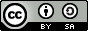# The thinking behind this tutorial

These practicals are designed to have an explanatary text, together with code examples. Note that all code examples have a light yellow background, and are boxed. Output from R is also shown, and text output is also boxed. Graphical output is shown ‘in line’. The idea is to copy the text in the boxes into a running version of R - you can use the output to see whether you are on the right track. Also, don’t be afraid to experiment - it may not always work, but for example playing around with some of the function parameters change some aspects of the analysis, or of the graphical presentation. Also, it is generally assumed that the code you type in from the boxes is actually entered in the same order as it appears here. Some of the later boxes depend on what was done earlier, and so skipping ahead might lead to errors. The help facility (putting a question mark in front of a command, for example ?plot, and hitting return) is also a good way to discover things…

# Analysis of Baltic Sea soils data from the mvoutlier package

Firstly, load all of the packages that r requires for this exercise.

# Load libraries
packages.wanted <- c("robustbase","mvoutlier","RColorBrewer","GWmodel")
for (package in packages.wanted) require(package,character.only=TRUE)

library(GWmodel)

If entering the above gives an error message, make sure the packages: robustbase, mvoutlier, RColorBrewer, GWmodel are all installed on your system.

Now, set up the data set (Data.1) used for this practical. This is a subset of the Baltic Soil Survey (BSS) data set (Reimann et al. 2000) from the mvoutlier package (retaining ten key variables and location information from the original).

data(bsstop)
Data.1 <- bsstop[,1:14]

Now, check the column names of this data set. There should be an ID, an X and Y location pair (called XCOO and YCOO where COO is short for coordinate), and 10 columns relating to the concentration of chemical compounds in the soil.

colnames(Data.1)
  "ID"      "CNo"     "XCOO"    "YCOO"    "SiO2_T"  "TiO2_T"  "Al2O3_T" "Fe2O3_T"
 "MnO_T"   "MgO_T"   "CaO_T"   "Na2O_T"  "K2O_T"   "P2O5_T" 

Next, we will be working with scaled data - that is we will replace the chemical concentration measurements by their z-scores. If you haven’t heared of z-scores before, if x is the original variable then $z = \frac{x - \bar{x}}{\textrm{sd}(x)}$ so that the variable is rescaled to have a mean value of zero, and a standard deviation of one. This means that we are measuring relative fluctuations in each individual chemical across geography, rather than measuring all concentrations on the same scale.

Data.1.scaled <- scale(as.matrix(Data.1[5:14])) # standardised data...
rownames(Data.1.scaled) <- Data.1[,1]

we also store the number of observation points in n1:

n1 <- length(Data.1.scaled[,1])
n1
 768

from this it may be seen that there are 768 sample locations. Using this data, compute principal components:

pca <- princomp(Data.1.scaled,cor=F,scores=T) # use covariance matrix to match the following...
pca\$loadings

Comp.1 Comp.2 Comp.3 Comp.4 Comp.5 Comp.6 Comp.7 Comp.8 Comp.9 Comp.10
SiO2_T   0.178 -0.587        -0.255  0.450  0.239 -0.423 -0.310  0.119
TiO2_T  -0.366 -0.182  0.300        -0.114        -0.454  0.673 -0.244
Al2O3_T -0.398 -0.213 -0.226        -0.136                       0.164  0.830
Fe2O3_T -0.399         0.274        -0.257               -0.184  0.747 -0.303
MnO_T   -0.316 -0.133  0.469  0.125  0.503  0.112  0.610
MgO_T   -0.379         0.170 -0.363 -0.256               -0.566 -0.546
CaO_T   -0.264  0.301 -0.338 -0.495  0.516 -0.439         0.103
Na2O_T  -0.322        -0.571                0.638  0.137  0.113        -0.355
K2O_T   -0.224 -0.489 -0.288  0.450        -0.540        -0.136 -0.172 -0.293
P2O5_T  -0.224  0.465         0.566  0.339  0.173 -0.465 -0.216

Comp.1 Comp.2 Comp.3 Comp.4 Comp.5 Comp.6 Comp.7 Comp.8 Comp.9 Comp.10
SS loadings       1.0    1.0    1.0    1.0    1.0    1.0    1.0    1.0    1.0     1.0
Proportion Var    0.1    0.1    0.1    0.1    0.1    0.1    0.1    0.1    0.1     0.1
Cumulative Var    0.1    0.2    0.3    0.4    0.5    0.6    0.7    0.8    0.9     1.0

The list of loadings, above, give the key components of variation in the soil data set. Recall - probably from several years ago ! - that the first principal component is an index, made from a weighted combination of the variables that gives the greatest variability in the study area. By looking at the values of the weights, we get a picture of what single characteristic accounts for most variability in the multivariate data. The second principal component finds the next most variable weighted index, given the further constraint that it is uncorrelated wiitgh the first, and so on for the other components. Looking down the columns show these weights. From this, we see that the first component contrasts the level of $$SiO_2$$ with all of the other compounds. In a nutshell, higher levels of $$SiO_2$$ correspond to larger particle size in the soil, which in turn suggests that the soil is more barren. The next component contrasts ( CaO ) and $$P_2O_5$$ with $$SiO_2$$, $$TiO_2$$, $$Al_2O_3$$, $$MnO$$ and $$K_2O$$. Note that cells in this table left blank correspond to ones with a weighting below 0.1 - a relatively low weighting. This suggests that $$Fe_2O_3$$, $$MgO$$ and $$Na_2O$$ do not play a notable role in the second component.

So the analysis tells us what the weightings are - but not what the geography actually is. A few lines of R will help to address this. Firstly the data set bss.background contains national borders for the study region:

data(bss.background)

Next we can draw a map. This is quite basic. Firstly plot the national borders - type='l' tells R that we want to draw lines. asp=1 makes sure the x- and y- axes are drawn to the same scale, so the map doesn’t appear ‘stretched’. All of the other options are used to switch off various details of the axes - although I am using plot to draw a map, it is essentially a graph-plotting command. It can be used for maps, but the default settings must be altered.

plot(bss.background,asp=1,type='l',xaxt='n',yaxt='n',xlab='',ylab='',bty='n',col='grey')